This section is Section 2 of 3.

Speed as well as accuracy is important in this section. Work quickly, or you might not finish the paper. There are no  penalties for incorrect responses, only marks for correct answers, so you should attempt all 27 questions. Each question is worth one mark.

You must complete the answers within the time limit. Calculators are NOT permitted.

Good Luck!

Note – if press “End Exam” you can access full worked solutions for all past paper questions

The diagram shows a kidney and its associated vessels from a healthy individual.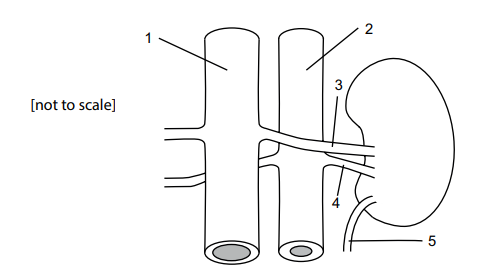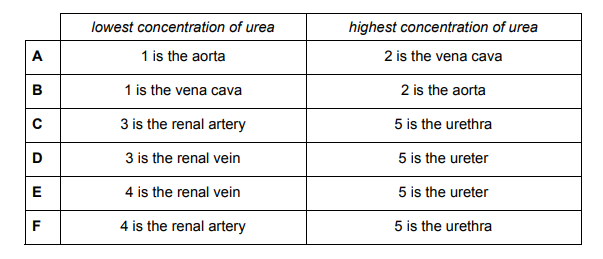1. Which row correctly identifies the vessels along with the concentration of urea they contain?
0
1

## Explanation

The correct answer is D: 3 is the renal vein, as this is the filtered blood obtained from the kidneys. During filtration, urea is removed from the blood, and the renal vein carries blood towards the heart. Hence, it has the lowest concentration of urea. 5 is the ureter which contains the highest concentration of urea. This is as, the filtrate contains urea which is filtered by the kidney to be excreted by the body.

Post Comment

Element X has the electronic structure 2, 8, 3.

1 The element is in Group 12, Period 3 of the Periodic Table.

2 The element reacts with oxygen to form a compound with the formula X2O3.

3 The element reacts with bromine to form a compound with the formula XBr3.

4 The atomic number of the element is 13.

5 The element is an alkali metal.

1
0

## Explanation

The correct answer is F: Statements 2, 3 and 4 are correct only.

Statement 1 is incorrect: A group of an element tells you the number of outer electrons, hence the element cannot be in group 12, as there are only 3 electrons in its outer shell. In a period, the number of electron shells are the same, and the period number is determined by the number of electron shells. Period 3 is correct, however, Group 12 is not.

Statement 5 is incorrect: Alkali metals occupy group 1 in the periodic table – they only have 1 electron in their outermost shell. Whereas, element X is in Group 3.

Statement 4 is correct: The atomic number is the number of protons, which is also the number of electrons, in the atom. Adding up the electrons in the 3 shells: 2 + 8 + 3 =13.

Statement 3 is correct: Bromine is in Group 7, hence has 7 electrons in its outermost shell. It needs 1 electron to complete its shell, which can hold a maximum of 8 electrons. Element X can give 3 electrons to gain a complete shell, which is composed of 8 electrons – below the outermost shell. Hence, X donates 1 electron each to 3 Bromine atoms, forming XBr3.

Statement 2 is correct: Oxygen is in Group 6, hence it has 6 electrons in its outermost shell – requiring 2 electrons to complete its shell. Element X has 3 electrons, and so one electron will remain spare! However, to resolve this issue, a lowest common multiple (LCM) of 2 and 3 must be found 6.

If there are 2X atoms, they have 6 electrons in total. If there are 3O atoms, they have 18 electrons in total. One X atom can give 2 electrons to each O atom. Hence, the formula is X2O3.

Post Comment

A student carries out an experiment to determine the density of the material from which two identical solid objects are made. She uses a balance and a measuring cylinder containing a fixed volume of liquid. The diagrams show different stages of her experiment, with some of the readings on the balance and some on the measuring cylinder.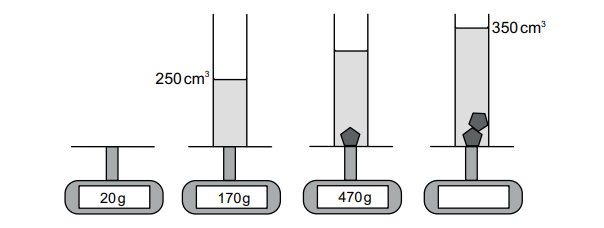3. Which calculation should be used to determine the density of the material from which the objects are made?
0
0

## Explanation

The correct answer is D: This is a displacement question. However, 2 solid objects are placed here. The

volume of displacement is: 350 – 250 = 100.

The mass of water initially: 170 – 20 = 150g ; The mass of the object (when singly placed): 470 – 170 = 300g.

When 2 objects added – mass: 2(300) = 600 mass overall: 600 + 170 = 770g

Difference in mass (from 0 solids 2 solids): 770 – 170 = 600

Density = Mass/Volume = 600 / 100 = 300 / 50

Post Comment

A straight line passes through the points P ( − 3, 3) and Q (6, 9).

4. Which one of the following is an equation of a straight line which is parallel to PQ?
0
0

## Explanation

The correct answer is A: An equation of a parallel line has the same gradient as the line PQ. Gradient:

y2y1/ x2x1 => yQyP/xQxP→ 9 – 3/6 – (-3) = 6/9 = 2/3

Hence, the gradient is 2/3. We are given no information on the coordinates of the parallel line, hence we cannot find the y intercept. Hence, the answer is y=2x/3 – 3

Post Comment

The diagram shows some of the stages of how a length of DNA can be removed from one organism and introduced into another organism.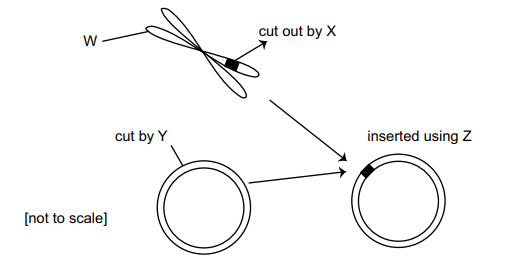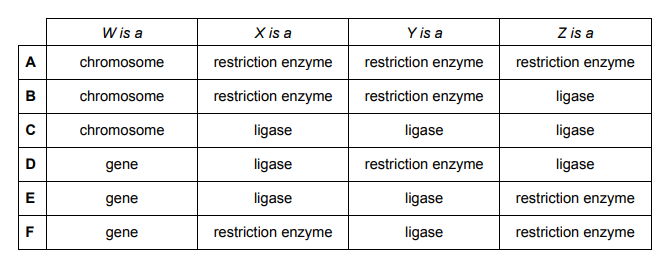5. Which row is correct?
0
0

## Explanation

The correct answer is B: W – Chromosome, which is shown by its characteristic ‘X’ shape during metaphase. X – restriction enzyme, as restriction enzymes are used to cut out a portion of DNA from the donor organism. Y – restriction enzyme, as the plasmid (shown by its circular structure) has to be cut up using the same restriction enzymes as used to cut the donor DNA, as they leave the same sticky ends. Z – ligase. This enzyme seals up and joins together the sticky ends of the plasmid – donor DNA – gene complex.

Post Comment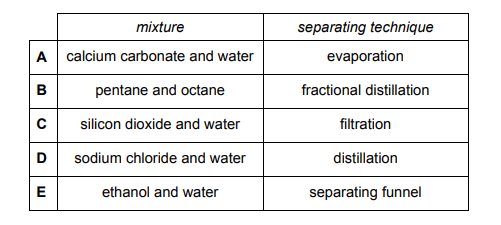6. Which one of the following mixtures could not be separated using the technique given?
0
0

## Explanation

Ethanol and water cannot be separated by a separating funnel. This is as, ethanol is soluble in water, as it forms hydrogen bonds with water molecules. Hence, distillation can separate them.

Post Comment

Nickel has an atomic number of 28. The mass numbers of four of its isotopes are 58, 60, 61 and 62.

Below are three statements about these isotopes of nickel.

1 All of them have the same chemical properties.

2 All of them have nuclei containing 28 protons.

3 One of them has a nucleus that contains 62 neutrons.

7. Which statement(s) is/are correct?
0
0

## Explanation

Statements 1 and 2 are correct. Isotopes have the same chemical properties, as they have the same atomic number. The atomic number dictates how many protons occupy the atom, which is also the same number as the electrons. If the electron number is the same, then the isotopes will have the same chemical properties. Statement 3 is incorrect: Beware, the question only gives mass numbers, not neutron numbers. Mass number = atomic number + number of neutrons. Hence, Statement 3 can be corrected by: 62 – 28 = 34. Hence, one of them has a nucleus containing 34 neutrons.

Post Comment

The mean mass of a group of N people is 75kg.

Jim, Karen and Leroy join this group, without anyone leaving; the new mean mass is 78kg.

The mean mass of Jim, Karen and Leroy is 90 kg.

8. What is the value of N?
0
1

## Explanation

The correct answer is B: 75 = Total mass/N J+K+L + Total Mass/N+3 = 78

J+K+L/3 = 90

Hence, J+K+L = 270

Substitute this into second equation: 270 + Total Mass /(N+3) = 78

Total Mass (from equation 1): 75N

270 + 75N/(N+3) = 78 78(N+3) = 270 + 75N 78N+234 = 270 + 75N 3N = 36

Hence, N = 12

Post Comment

The following statements are features of an enzyme from a healthy human.

1 It works at an optimum pH below 4.

2 It digests a substrate into amino acids.

3 It works at an optimum temperature of approximately 37°C.

9. Which enzyme has these features?
0
0

## Explanation

These statements all apply to protease. Protease works in optimally acidic conditions. The lower the pH, the greater the acidity of the solution. The hydrochloric (HCl) acid in the stomach ranges from pH 1.5 – 3.5 for protease to catalyse the breakdown of proteins. Protease digests proteins into amino acids. Protease, like all digestive enzymes, have an optimum temperature of 37°C.

Post Comment

Mohr’s Salt is a common laboratory reagent. Use the information of the most abundant isotopes below to calculate the formula mass, Mr, of the hydrated salt.

Formula of Mohr’s Salt: (NH₄)₂Fe(SO₄)₂.6H₂O

Most abundant isotopes: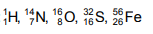10.
0
0

## Explanation

To calculate the mass, the mass numbers of all elements are needed. Hence (in order of the formula):

2(14 + 4(1)) + 56 + 2(32 + 4(16)) + 6( 2(1) + 16) = 36 + 56 + 192 + 108 = 392

Post Comment

An AC voltage is induced in a coil of wire when it is rotated in a magnetic field.

This voltage can be displayed on the screen of an instrument called an oscilloscope, which shows the variation of the induced voltage on the y axis plotted against time on the x-axis.

The oscilloscope trace below is the result of rotating a coil at a constant speed in a uniform magnetic field.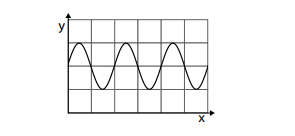11. Which one of the following traces would result from rotating the coil at a faster constant speed, whilst keeping all other conditions unchanged? (The scales on the axes do not change.)
0
0

## Explanation

Rotating the coil at a faster constant speed, increases the induced voltage produced. Hence, it leads to an increase in amplitude of the oscilloscope graph. This is as, a large voltage corresponds to the y axis. As the coil rotates at a faster speed, voltage is induced at a faster rate, hence the time period decreases, as voltage is being induced faster due to faster relative motion. This increases the frequency of the induced voltage, hence more waves are observed on the oscilloscope graph.

Post Comment

The diagram represents the circular cross-section of an artery with external diameter d of 1.6 cm. The thickness t of the artery wall is 1mm.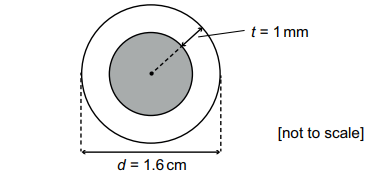12. What is the internal cross-sectional area of the artery (shaded in the diagram)?
0
0

## Explanation

To find the shaded area, the formula: A =  π

r2is needed. We are given the diameter of the artery as 1.6cm, with the extension from the artery wall being 1mm. Be careful of units here – convert all into mm, as the answers are in mm. Hence, the diameter of the artery is 16mm. In order to get the radius of the artery wall, 2mm must be subtracted from the artery diameter – 2mm as t=1mm, which extends in both directions from the diameter.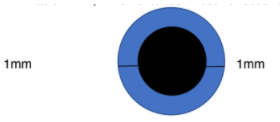Hence, 16-2=14. Radius=Diameter/2, hence r=7. A= π

(7)2=49 π

Post Comment

1 carbon dioxide

2 glucose

3 lactic acid

13. Which of the following molecules are involved in both aerobic and anaerobic respiration in a healthy human?
0
0

## Explanation

Glucose is involved in both aerobic and anaerobic respiration. Carbon dioxide and water are products of aerobic respiration, whereas lactic acid is a product of anaerobic respiration. Hence, Statements 1 and 3 are incorrect, as they don’t apply to both respiratory processes; glucose is required for both processes to occur.

Post Comment

Identify the correct products of electrolysis of the following electrolytes.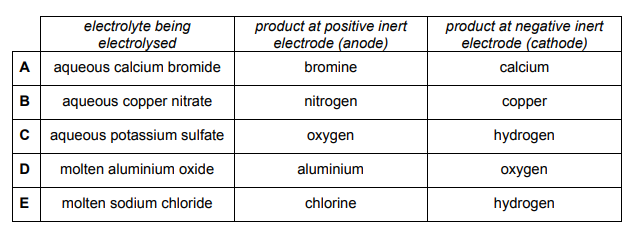14.
0
0

## Explanation

The electrolysis of an aqueous solution of potassium sulfate using inert electrodes produces hydrogen at the cathode and oxygen at the anode and a neutral solution of sodium sulfate remains unaltered by the electrolysis. This is as inert electrodes are used. Hence, it cannot be A, B, or D as they produce a metal at the electrodes. For this to occur, the electrodes must be of that metal i.e. Copper electrodes with CuSO₄ would lead to copper being deposited on the electrodes.

Anode half equation (At the positive electrode, negatively charged ions lose electrons. This is oxidation.) : 2H₂O O₂ + 4H+ + 4e-

Hence, oxygen is formed at the anode.

Cathode half equation (At the negative electrode, positively charged ions gain electrons. This is reduction.) : 4H₂O + 4e- 2H₂ + 4OH-

Hence, hydrogen is formed at the cathode.

Post Commentfat fio Medicmind Tutor

Mon, 01 Nov 2021 00:39:46

I scored really well and I appreciate this site for helping me in my BMAT endeavours. I now study in Cambridge thanks to this website!skinny fio Medicmind Tutor

Mon, 01 Nov 2021 22:44:53

nice

The diagram represents a satellite communication link between two points on Earth. The distances between the transmitting station, the satellite and the receiving station are shown. The frequency of the waves used for the link can be taken as 1.5 × 10¹⁰ Hz, and the speed of
light as 3.0 × 10⁸ m/ s.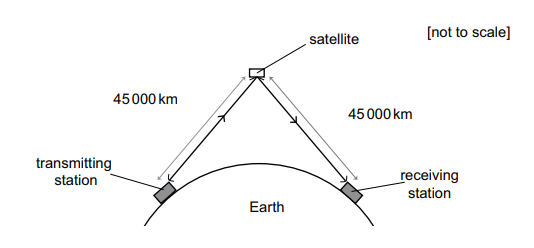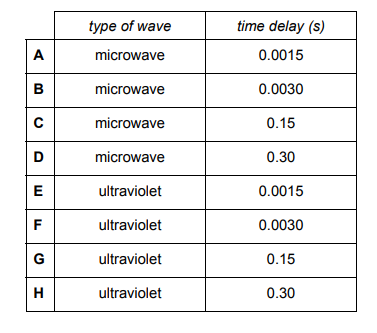15. What type of wave is used in such a link, and what is the time delay between a signal being transmitted and then being received at the receiving station?
0
0

## Explanation

Microwaves are used for satellite communication.

Total distance = 2 ( 45000 x 1000) = 9 X 107m

Speed = Distance/Time

9 X 107/(3 X 108) = 0.30s

The frequency given is irrelevant, as it does not partake in the calculation.

Post Comment

The diagram shows a quadrilateral PQRS.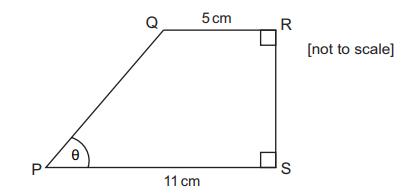16. Given that tan θ = 4 / 3 , what is the area of the quadrilateral PQRS?
0
0

## Explanation

To find the area, we must divide the shape into a right angled triangle PQX (where X is the vertex of this triangle on the line PS), and a rectangle QRSX.

The area of the triangle = 1⁄2 bh; The opposite to θ is the height (4) and the adjacent to θ is the base (3). However, the actual length of PX is 11-5=6. Remember tan is a ratio of sides, hence the ratio of O:A is 4:3. The actual length is O:6, hence O = 8 as the factor is 2.

Area of triangle = 1⁄2 x 6 x 8 = 24

Area of rectangle = 8 x 5 = 40

Area of quadrilateral = area of triangle + area of rectangle = 24 + 40 = 64

Post Comment

1 A new allele is formed coding for a protease enzyme that works more efficiently.

2 A new allele is formed coding for a protease enzyme that works less efficiently.

3 A new allele is formed coding for a non-functional protein that has no effect on the cell.

4 A new allele is formed coding for a non-functional protein that has a negative effect on the cell.

17. Which of the following statements could describe the result of a single mutation of a gene coding for a protease enzyme in a fertilised human egg cell?
0
0

## Explanation

All 4 Statements are correct. A mutation leads to a change in the base sequence of amino acids, which in turn leads to a change in the structure of the protein that is produced. Each triplet of bases codes for one amino acid. The order of bases determines the sequence of amino acids, thus dictating which protein is coded for. A mutation can occur through: substitution (in a base is substituted by another base) , deletion (a base is not added to the sequence) and addition (an extra base is added in the sequence) of bases. These all include the possibilities of either a functional/non functional protein being coded for, depending upon which base is deleted /added/ substituted. Hence, both Statements 3 and 4 are correct, as enzymes are proteins (including protease), and the mutation can have a negative, positive or no effect on the non functional protein (the protease enzyme may not perform its role, but it may lead to other consequences, or it may not affect cellular activity at all). Statements 1 and 2 are correct, as the change of protease structure may cause it to bind more tightly to protein substrates, or loosely; hence, this determines the efficiency of the protease.

Post Comment

What volume of a 0.10mol dm–³ solution of NaOH is needed to neutralise 30 cm³ of a 0.20 mol dm–³ aqueous solution of a diprotic acid?

18.
2
3

## Explanation

As the acid is diprotic, and NaOH neutralises the acid, 2 moles of NaOH are needed.

n = c x v x 10-3 n (Diprotic) = 30 x 0.2 x 10-3 = 0.006

As 2 NaOH, n(NaOH) = 2 x 0.006 = 0.012

For base (NaOH): v = (n/c) x 1000 v = (0.012/0.1) x 1000 = 120 cm3

Post Comment

A defibrillator provides an electric shock to a heart to restore its normal rhythm. The energy to do this is 125 J, at a steady voltage of 500V, for a time of 10 milliseconds.

19. What is the current during this process?
0
0

## Explanation

Energy = Power X Time = Current X Voltage X Time

125 = 500 x 10 x 10-3 x current

Current = 125/(500 x 10 x 10-3) = 250

Post Comment

Rearrange the formula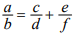to make f the subject.

20.
0
0

## Explanation

f needs to be made the subject.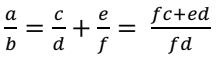Firstly, add here, we have cross multiplied fc and ed, as the lowest common multiple (LCM) of the denominator is fd.

Then, multiply both sides by fd in order to factorise later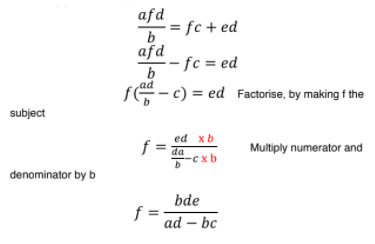Post Comment

1 asexual reproduction

2 growth of a cell

3 repair of cells

4 stem cell division

21. Which statements describe a role of mitosis?
1
3

## Explanation

Statement 1 and 4 are correct, as they involve division. Mitosis is the process of cell division, whereby 2 daughter cells are produced which have the same number of chromosomes, hence are genetically identical. Asexual reproduction produces genetically identical offspring, through mitosis (i.e. bacterial reproduction). Stem cells also produce genetically identical stem cells through mitosis. Be careful – mitosis makes new cells for growth, repair and replacement (i.e. cell replacement in humans, or reproduction in microorganisms). Statement 2 is incorrect as it mentions growth of a cell – mitosis produces cells for growth of a tissue, which is composed of many cells. Statement 3 is incorrect too, as a tissue is repaired through the production of cells by mitosis – not the cell itself.

Post Comment

Calcium carbonate reacts with hydrochloric acid. The reaction gives off carbon dioxide gas.

Line X on the graph shows the volume of carbon dioxide formed against time when 100 cm³ of 1.0 mol dm–³ of hydrochloric acid reacts with calcium carbonate chips at 20°C. There was an excess of calcium carbonate chips.

CaCO3 + 2HCl → CaCl2 + CO2 + H2O(l)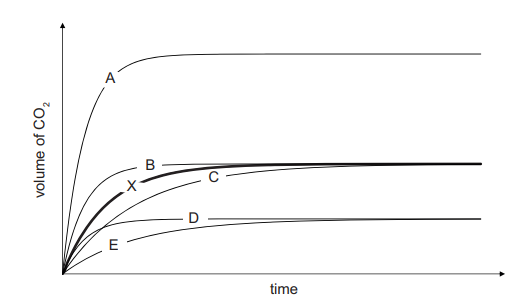22. Which line best represents the volume of carbon dioxide formed against time when the reaction is repeated with 50 cm³ of 2.0 mol dm–³ of hydrochloric acid reacting with excess calcium carbonate chips at 20°C?
0
0

## Explanation

moles = concentration x volume x 10-3

n (X): 100 x 1 x 10-3 = 0.1

mole ratio of 2HCl : CO2 = 2:1

n(B): 50 x 2 x 10-3 = 0.1

The moles for both conditions are the same, hence indicating that the final volume of CO2 produced is constant – the same. Hence, the line has to reach the same endpoint as X – it cannot be D, E or A. It’s either C or B. However, the time taken to reach the end point of X (the rate of reaction) will differ. The concentration of HCl is higher in the second experiment, hence this increases the rate of reaction. This is as, a higher concentration of HCl molecules increase the likelihood of successful collisions with calcium carbonate chips, as they are more densely occupied in a small volume. Hence, it cannot be C, as the time taken is longer to reach the end point. Hence, it has to be B, as the time taken is smaller.

Post Comment

An object that has a weight of 15N on Earth is taken to a planet where it has a weight of 3.0 N. The planet has no atmosphere.

(Take the gravitational field strength g on Earth to be 10N/ kg.)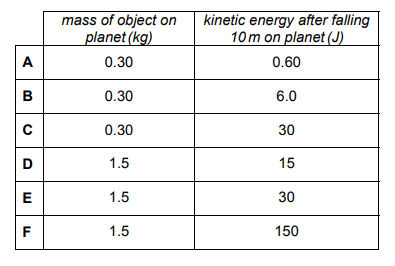23. Which line in the table correctly shows the mass of the object on the planet, and the kinetic energy it gains after falling from rest near the surface of the planet through a vertical distance of 10 m?
0
1

## Explanation

W=mg – Mass of the object does not change on any planet, only the weight changes, due to the different gravitational strengths.

M = W/g hence, on Earth: 15/10 = 1.5 kg.

Kinetic energy gained on planet = gravitational energy lost on planet Mgh = gravitational energy lost (as no velocity is provided, cannot calculate kinetic energy directly, hence we use the conservation of energy). W = mg, hence use 3.0N as mg. Hence, 3 x 10 = 30J

Post Comments Medicmind Tutor

Tue, 28 Jun 2022 14:28:16

bruh could not you say moon instead of planet so its not mistaken from earth

Some blood is found at a crime scene. The police know that it belongs to the one criminal involved.

A person’s red blood cells can have type A antigens, type B antigens, both types or neither type.

In a population:

45% of people have type A antigens but not type B

9% of people have type B antigens but not type A

43% of people have neither type of antigen

3% of people have both types of antigen

An antibody test shows that there are type B antigens present in the red blood cells at the crime scene.

24. What is the probability that the criminal’s red blood cells have both type A and type B antigens?
1
1

## Explanation

Defining: P(AB’) = 0.45, P(BA’) = 0.09

P(A’B’)=0.43, P(AB)=0.03.

Rewrite probabilities as equations:

A(1-B) = 0.45

A-AB=0.45

B(1-A) = 0.09 B-AB=0.09

(1-A)(1-B) = 0.43 AB – (A+B)+1=0.43

AB = 0.03

Hence, substituting AB = 0.03, A-0.03 = 0.45 , hence A = 0.48

B – 0.03 = 0.09 ; B = 0.12

To check: 0.03 – (0.12 + 0.48) + 1 = 1.03 – 0.60 = 0.43

Given the presence of Type B antigens, the probability off both types need to be found. This is, the probability of both/given

Hence, 0.03/0.12 = 1⁄4

Post Comment

An experiment was carried out to investigate a gene for coat colour in mice.

The diagram shows the results of three crosses between different mice, producing three different families, P, Q and R.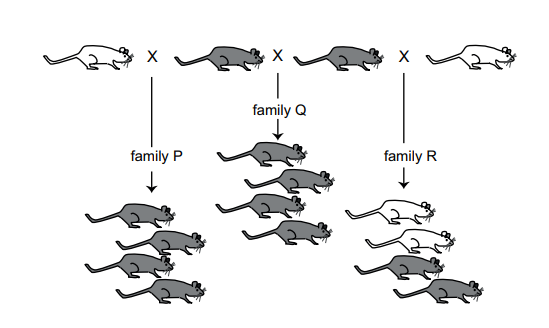25. What is the maximum possible number of heterozygous mice shown in the diagram?
0
0

## Explanation

This question asks for the maximum number of heterozygous mice.

We don’t know whether the characteristic Grey is dominant, or whether White is dominant. Hence, grey mice are either dominant or recessive, and the same applies for White mice.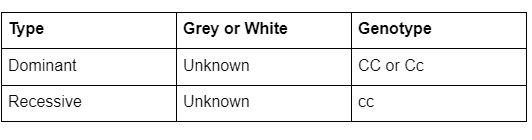To get the smallest number of heterozygous mice, we can assume that all dominant mice are heterozygous dominant (Cc), not homozygous dominant (CC).

All of the recessive mice are homozygous recessive (cc), as you cannot get heterozygous recessive.

There are 12 grey mice, and 4 white mice. Hence, it seems sensible to make the white colour recessive as the recessive mice are homozygous. We want to maximise heterozygous. Hence, at maximum, there are 12 heterozygous mice.

Post Comment

The gases X and Y react with each other to produce gas Z according to the equation:

2X(g) + Y(g) → 2Z(g)

100 cm³ of X was mixed with 10 cm³ of Y, in a freely moving gas syringe sealed with a rubber cap. The reaction went to completion. All volumes were measured at the same temperature and pressure.

26. What is the final volume of gas in the syringe?
0
0

## Explanation

2X + Y 2Z. There is a 1:1 mole ratio between X and Z, and as moles are proportional to volume (in n=cv), the volume of X and Z must be the same. The question states that 2X and Y react together to form a compound which has the same moles as X. Hence, the final volume of Z is 100 cm3 too.

Post CommentAnonymous Medicmind Tutor

Mon, 01 Nov 2021 15:46:53

Please could someone explain the logic? Surely the final volume of Z is 20cm3? If moles are proportional to volume, Y is the limiting reagent: so 20 moles of X and 10 moles of Y make 20 moles of Z. Final volume = 20cm3 of Z + 80cm3 of unreacted Y = 100 cm3s Medicmind Tutor

Tue, 28 Jun 2022 14:29:23

Light travels through glass at a speed which is 2 / 3 of its speed through air.

Light travels through water at a speed which is 3 / 4 of its speed through air.

A ray of light has a wavelength of 360 nm when travelling through water.

27. What is the wavelength of this ray of light when travelling through glass?
0
0

## Explanation

Frequency does not change when a light travels through a medium. Hence, the frequency of light through water should be

calculated first: c=fλ ; 3/4 x (3 X 108) = 2.25 x 108 2.25 x 108 = (360 x 10-9) x f

f = 2.25 x 108 / 360 x 10-9 = 6.25 x 1014

Glass: 2/3 x (3 X 108) = 2 x 108 2 x 108 / 6.25 x 1014 = 3.2 x 10-7 = 320 nm

Post Comment

## Instructions

Below is a summary of your answers. You can review your questions in three (3) different ways.

The buttons in the lower right-hand corner correspond to these choices:

2. Review questions that are incomplete.
3. Review questions that are flagged for review. (Click the 'flag' icon to change the flag for review status.)

You may also click on a question number to link directly to its location in the exam.

## Instructions

This review section allows you to view the answers you made and see whether they were correct or not. Each question accessed from this screen has an 'Explain Answer' button in the top left hand side. By clicking on this you will obtain an explanation as to the correct answer.

At the bottom of this screen you can choose to 'Review All' answers, 'Review Incorrect' answers or 'Review Flagged' answers. Alternatively you can go to specific questions by opening up any of the sub-tests below.

x

## When should we call you?

It would be great to have a 15m chat to discuss a personalised plan and answer any questions

## What time works best for you? (UK Time)

Pick a time-slot that works best for you ?

Submit

Submit